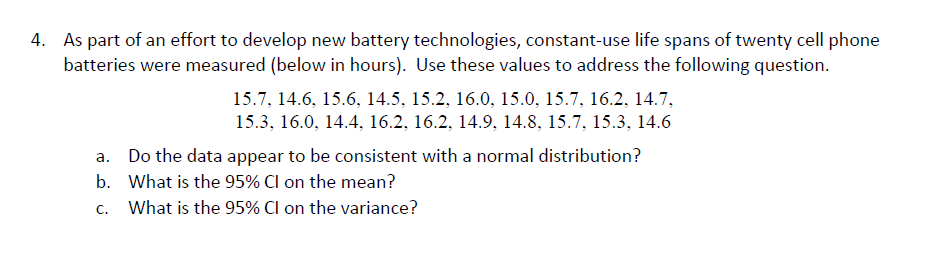# As part of an effort to develop new battery technologies, constant-use life spans of twenty cell phonebatteries were measured (below in hours). Use these values to address4.the following question15.7. 14.6. 15.6. 14.5. 15.2. 16.0. 15.0. 15.7. 16.2. 14.7,15.3. 16.0, 14.4, 16.2, 16.2. 14.9, 14.8.15.7, 15.3, 14.6a.b,c.Do the data appear to be consistent with a normal distribution?what is the 95% CI on the mean?What is the 95% CI on the variance?

Question
15 viewshelp_outlineImage TranscriptioncloseAs part of an effort to develop new battery technologies, constant-use life spans of twenty cell phone batteries were measured (below in hours). Use these values to address 4. the following question 15.7. 14.6. 15.6. 14.5. 15.2. 16.0. 15.0. 15.7. 16.2. 14.7, 15.3. 16.0, 14.4, 16.2, 16.2. 14.9, 14.8. 15.7, 15.3, 14.6 a. b, c. Do the data appear to be consistent with a normal distribution? what is the 95% CI on the mean? What is the 95% CI on the variance? fullscreen
check_circle

Step 1

a.

Check whether the given data appear to be consistent with normal distribution or not:

The data represents the constant use life spans for a random sample of 20 cell phone batteries.

The conditions that describe the consistency of a dataset with normal distribution using the normal probability plot are given below:

• The data points should form an approximate straight line. Departure of data points from straight line indicates departure from normality.
• The plot should not show any outliers.

Here, normal probability plot is constructed using the MINITAB software.

Software Procedure:

Step-by-step procedure to draw the normal probability plot using the MINITAB software:

• Choose Graph > Probability plot.
• Select Simple, and then click OK.
• In Graph variables, enter the column of life spans.
• Click

Output of histogram for the sample dataset of life spans is obtained from the MINITAB software is given below:

Step 2

Comment on the consistent of the data with normal distribution.

From the normal probability plot it is observed that the data points fall near the straight line. This implies that the dataset is approximately normally distributed.

Since, none of the points are far away from the remaining data points. The data does not show any outliers.

Thus, all the conditions are also satisfied.

Hence, it can be concluded that the data appear to be consistent with normal distribution.

Step 3

b.

Construct the 95% confidence interval for the mean life span of cell phone batteries:

Denote the population mean life span of cell phone batteries as μ

Here, 95% confidence interval for the mean life span of cell phone batteries is obtained using MINITAB software.

Software Procedure:

Step by step procedure to obtain the 95% confidence interval to estimate mean life span of cell phone batteries using the MINITAB software:

• Choose Stat > Basic Statistics > 1 sample t.
• Choose one or more samples in a column.
• Enter the column of life spans.
• C...

### Want to see the full answer?

See Solution

#### Want to see this answer and more?

Solutions are written by subject experts who are available 24/7. Questions are typically answered within 1 hour.*

See Solution
*Response times may vary by subject and question.
Tagged in
MathStatistics

### Other